The two screen shots below show the interface for single digit addition. Your child clicks the four answers until the correct answer is found. The upper image shows all four answers clicked. The "Next Problem" overlay is not shown here.

Note: the user interface for single digit subtraction & multiplication is essentially the same as addition.This image shows how the problem is presented after the right answer has been clicked. The optional "Next Problem" overlay pauses the move to the next problem allowing your child to review the problem just solved.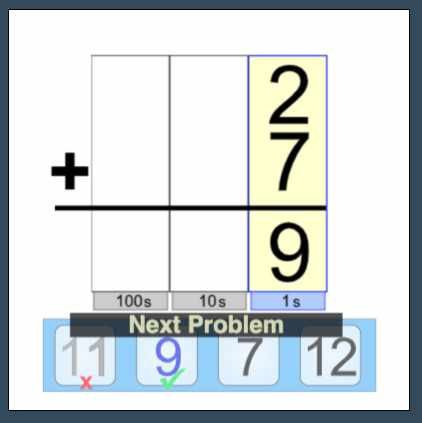Two Digit Subtraction with Borrowing

The screen shots below are an example of how RMM walks your child through the process of solving multi-digit problems. At the start of this problem, RMM shows the optional alert saying that borrowing is needed.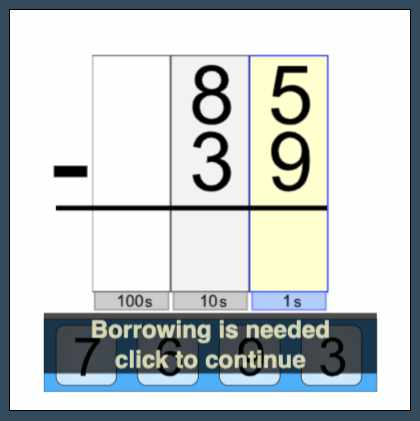Once the "borrowing is needed" note is cleared, RMM show the problem with all the borrows indentified. The optional borrowing note explantion is shown below the equation.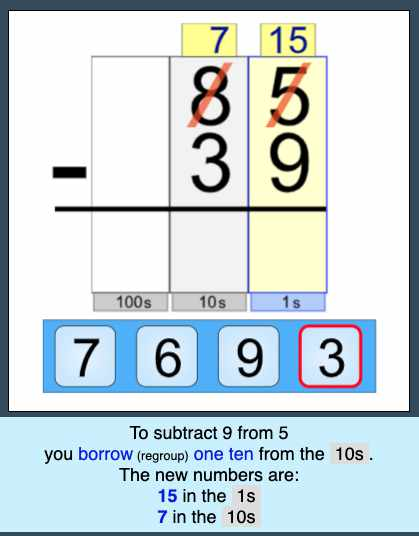After the right answer for the ones column has been clicked, RMM shows the optional popup identifying that the tens column is next to be solved.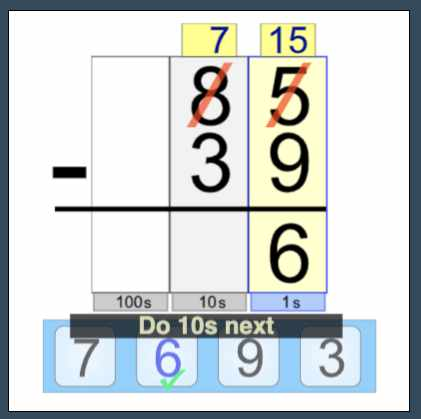As with single digit addition above, once the correct answer is provided for the tens column, RMM pauses and shows the "Next Problem" prompt allowing your child to review the equation just solved.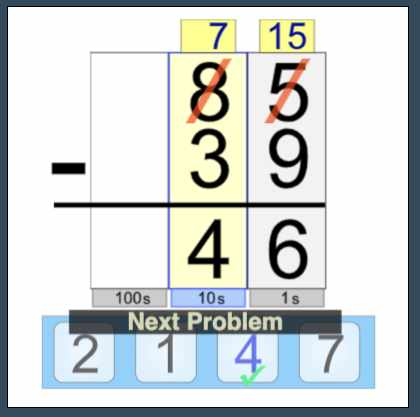The example above showing how RMM presents the two-digit subtraction problem characterizes RMM's algorithmic approach once one-digit arithmetic has been mastered. The same approach is used for: Two & three digit addition, Two & Three digit subtraction, Two digit multiplication and, Long Division. Of course the process steps will be customized for each different algorithmic step. To investigate these types of problems, run the full app using the link on the main RMM page.

The end